# 6.4 Conductors in electrostatic equilibrium  (Page 5/9)

 Page 8 / 9

The infinite slab between the planes defined by $z=\text{−}a\text{/}2$ and $z=a\text{/}2$ contains a uniform volume charge density $\rho$ (see below). What is the electric field produced by this charge distribution, both inside and outside the distribution?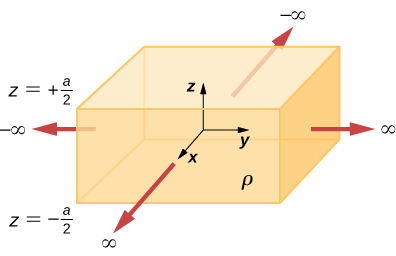Construct a Gaussian cylinder along the z -axis with cross-sectional area A .
$|z|\ge \frac{a}{2}\phantom{\rule{0.2em}{0ex}}{q}_{\text{enc}}=\rho Aa,\phantom{\rule{0.2em}{0ex}}\text{Φ}=\frac{\rho Aa}{{\epsilon }_{0}}⇒E=\frac{\rho a}{2{\epsilon }_{0}}$ ,
$|z|\le \frac{a}{2}\phantom{\rule{0.2em}{0ex}}{q}_{\text{enc}}=\rho A2z,\phantom{\rule{0.2em}{0ex}}E\left(2A\right)=\frac{\rho A2z}{{\epsilon }_{0}}⇒E=\frac{\rho z}{{\epsilon }_{0}}$

A total charge Q is distributed uniformly throughout a spherical volume that is centered at ${O}_{1}$ and has a radius R . Without disturbing the charge remaining, charge is removed from the spherical volume that is centered at ${O}_{2}$ (see below). Show that the electric field everywhere in the empty region is given by

$\stackrel{\to }{\text{E}}=\frac{Q\stackrel{\to }{\text{r}}}{4\pi {\epsilon }_{0}{R}^{3}},$

where $\stackrel{\to }{\text{r}}$ is the displacement vector directed from ${O}_{1}\phantom{\rule{0.2em}{0ex}}\text{to}\phantom{\rule{0.2em}{0ex}}{O}_{2}.$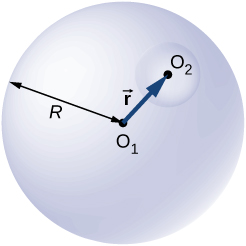A non-conducting spherical shell of inner radius ${a}_{1}$ and outer radius ${b}_{1}$ is uniformly charged with charged density ${\rho }_{1}$ inside another non-conducting spherical shell of inner radius ${a}_{2}$ and outer radius ${b}_{2}$ that is also uniformly charged with charge density ${\rho }_{2}$ . See below. Find the electric field at space point P at a distance r from the common center such that (a) $r>{b}_{2},$ (b) ${a}_{2} (c) ${b}_{1} (d) ${a}_{1} and (e) $r<{a}_{1}$ .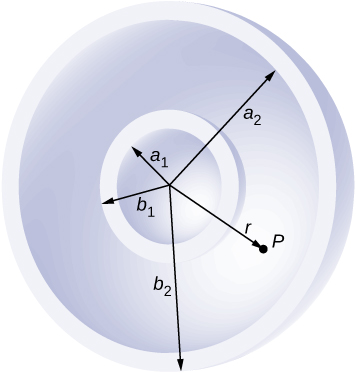a. $r>{b}_{2}\phantom{\rule{0.2em}{0ex}}E4\pi {r}^{2}=\frac{\frac{4}{3}\pi \left[{\rho }_{1}\left({b}_{1}^{3}-{a}_{1}^{3}\right)+{\rho }_{2}\left({b}_{2}^{3}-{a}_{2}^{3}\right)}{{\epsilon }_{0}}⇒E=\frac{{\rho }_{1}\left({b}_{1}^{3}-{a}_{1}^{3}\right)+{\rho }_{2}\left({b}_{2}^{3}-{a}_{2}^{3}\right)}{3{\epsilon }_{0}{r}^{2}}$ ;
b. ;
c. ;
d. ; e. 0

Two non-conducting spheres of radii ${R}_{1}$ and ${R}_{2}$ are uniformly charged with charge densities ${\rho }_{1}$ and ${\rho }_{2},$ respectively. They are separated at center-to-center distance a (see below). Find the electric field at point P located at a distance r from the center of sphere 1 and is in the direction $\theta$ from the line joining the two spheres assuming their charge densities are not affected by the presence of the other sphere. ( Hint: Work one sphere at a time and use the superposition principle.)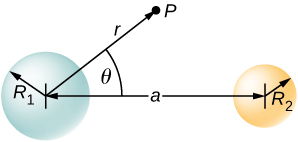A disk of radius R is cut in a non-conducting large plate that is uniformly charged with charge density $\sigma$ (coulomb per square meter). See below. Find the electric field at a height h above the center of the disk. $\text{(}h\phantom{\rule{0.2em}{0ex}}>>\phantom{\rule{0.2em}{0ex}}R,h\phantom{\rule{0.2em}{0ex}}<<\phantom{\rule{0.2em}{0ex}}l\phantom{\rule{0.2em}{0ex}}\text{or}\phantom{\rule{0.2em}{0ex}}w\text{).}$ ( Hint: Fill the hole with $±\sigma .\right)$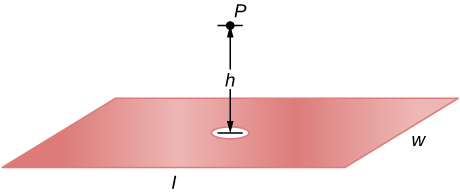Electric field due to plate without hole: $E=\frac{\sigma }{2{\epsilon }_{0}}$ .
Electric field of just hole filled with $\text{−}\sigma \phantom{\rule{0.2em}{0ex}}E=\frac{\text{−}\sigma }{2{\epsilon }_{0}}\left(1-\frac{z}{\sqrt{{R}^{2}+{z}^{2}}}\right)$ .
Thus, ${E}_{\text{net}}=\frac{\sigma }{2{\epsilon }_{0}}\phantom{\rule{0.2em}{0ex}}\frac{h}{\sqrt{{R}^{2}+{h}^{2}}}$ .

Concentric conducting spherical shells carry charges Q and – Q , respectively (see below). The inner shell has negligible thickness. Determine the electric field for (a) $r (b) $a (c) $b and (d) $r>c.$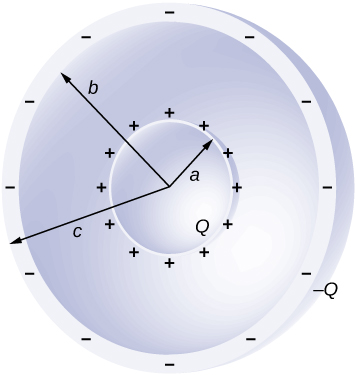Shown below are two concentric conducting spherical shells of radii ${R}_{1}$ and ${R}_{2}$ , each of finite thickness much less than either radius. The inner and outer shell carry net charges ${q}_{1}$ and ${q}_{2},$ respectively, where both ${q}_{1}$ and ${q}_{2}$ are positive. What is the electric field for (a) $r<{R}_{1};$ (b) ${R}_{1} and (c) $r>{R}_{2}?$ (d) What is the net charge on the inner surface of the inner shell, the outer surface of the inner shell, the inner surface of the outer shell, and the outer surface of the outer shell?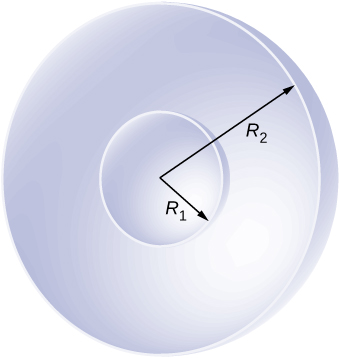a. $E=0$ ; b. $E=\frac{{q}_{1}}{4\pi {\epsilon }_{0}{r}^{2}}$ ; c. $E=\frac{{q}_{1}+{q}_{2}}{4\pi {\epsilon }_{0}{r}^{2}}$ ; d. $0\phantom{\rule{0.2em}{0ex}}{q}_{1}-{q}_{1},\phantom{\rule{0.2em}{0ex}}{q}_{1}+{q}_{2}$

A point charge of $q=5.0\phantom{\rule{0.2em}{0ex}}×\phantom{\rule{0.2em}{0ex}}{10}^{-8}\phantom{\rule{0.2em}{0ex}}\text{C}$ is placed at the center of an uncharged spherical conducting shell of inner radius 6.0 cm and outer radius 9.0 cm. Find the electric field at (a) $r=4.0\phantom{\rule{0.2em}{0ex}}\text{cm}$ , (b) $r=8.0\phantom{\rule{0.2em}{0ex}}\text{cm}$ , and (c) $r=12.0\phantom{\rule{0.2em}{0ex}}\text{cm}$ . (d) What are the charges induced on the inner and outer surfaces of the shell?

## Challenge problems

The Hubble Space Telescope can measure the energy flux from distant objects such as supernovae and stars. Scientists then use this data to calculate the energy emitted by that object. Choose an interstellar object which scientists have observed the flux at the Hubble with (for example, Vega http://adsabs.harvard.edu/abs/2004AJ....127.3508B ), find the distance to that object and the size of Hubble’s primary mirror, and calculate the total energy flux. ( Hint: The Hubble intercepts only a small part of the total flux.)

Given the referenced link, using a distance to Vega of $237\phantom{\rule{0.2em}{0ex}}×\phantom{\rule{0.2em}{0ex}}{10}^{15}$ m http://webviz.u-strasbg.fr/viz-bin/VizieR-5?-source=I/311&HIP=91262 and a diameter of 2.4 m for the primary mirror, http://ntrs.nasa.gov/archive/nasa/casi.ntrs.nasa.gov/19910003124.pdf we find that at a wavelength of 555.6 nm, Vega is emitting $1.1\phantom{\rule{0.2em}{0ex}}×\phantom{\rule{0.2em}{0ex}}{10}^{25}\phantom{\rule{0.2em}{0ex}}\text{J/s}$ at that wavelength. Note that the flux through the mirror is essentially constant.

Re-derive Gauss’s law for the gravitational field, with $\stackrel{\to }{g}$ directed positively outward.

An infinite plate sheet of charge of surface charge density $\sigma$ is shown below. What is the electric field at a distance x from the sheet? Compare the result of this calculation with that of worked out in the text.The symmetry of the system forces $\stackrel{\to }{\text{E}}$ to be perpendicular to the sheet and constant over any plane parallel to the sheet. To calculate the electric field, we choose the cylindrical Gaussian surface shown. The cross-section area and the height of the cylinder are A and 2 x , respectively, and the cylinder is positioned so that it is bisected by the plane sheet. Since E is perpendicular to each end and parallel to the side of the cylinder, we have EA as the flux through each end and there is no flux through the side. The charge enclosed by the cylinder is $\sigma A,$ so from Gauss’s law, $2EA=\frac{\sigma A}{{\epsilon }_{0}},$ and the electric field of an infinite sheet of charge is
$E=\frac{\sigma }{2{\epsilon }_{0}},$ in agreement with the calculation of in the text.

A spherical rubber balloon carries a total charge Q distributed uniformly over its surface. At $t=0$ , the radius of the balloon is R . The balloon is then slowly inflated until its radius reaches 2 R at the time ${t}_{0}.$ Determine the electric field due to this charge as a function of time (a) at the surface of the balloon, (b) at the surface of radius R , and (c) at the surface of radius 2 R . Ignore any effect on the electric field due to the material of the balloon and assume that the radius increases uniformly with time.

Find the electric field of a large conducting plate containing a net charge q . Let A be area of one side of the plate and h the thickness of the plate (see below). The charge on the metal plate will distribute mostly on the two planar sides and very little on the edges if the plate is thin.There is Q /2 on each side of the plate since the net charge is Q : $\sigma =\frac{Q}{2A}$ ,
$\underset{S}{\oint }\stackrel{\to }{\text{E}}·\stackrel{^}{\text{n}}\phantom{\rule{0.2em}{0ex}}dA=\frac{2\sigma \text{Δ}A}{{\epsilon }_{0}}⇒{E}_{P}=\frac{\sigma }{{\epsilon }_{0}}=\frac{Q}{{\epsilon }_{0}2A}$

Is it possible to find the magnetic field of a circular loop at the centre by using ampere's law?
Is it possible to find the magnetic field of a circular loop at it's centre?
yes
Brother
The density of a gas of relative molecular mass 28 at a certain temperature is 0.90 K kgmcube.The root mean square speed of the gas molecules at that temperature is 602ms.Assuming that the rate of diffusion of a gas in inversely proportional to the square root of its density,calculate the density of
A hot liquid at 80degree Celsius is added to 600g of the same liquid originally at 10 degree Celsius. when the mixture reaches 30 degree Celsius, what will be the total mass of the liquid?
what is electrostatics
Study of charges which are at rest
himanshu
Explain Kinematics
Two equal positive charges are repelling each other. The force on the charge on the left is 3.0 Newtons. Using your notes on Coulomb's law, and the forces acting on each of the charges, what is the force on the charge on the right?
Using the same two positive charges, the left positive charge is increased so that its charge is 4 times LARGER than the charge on the right. Using your notes on Coulomb's law and changes to the charge, once the charge is increased, what is the new force of repulsion between the two positive charges?
Nya
A mass 'm' is attached to a spring oscillates every 5 second. If the mass is increased by a 5 kg, the period increases by 3 second. Find its initial mass 'm'
a hot water tank containing 50,000g of water is heated by an electric immersion heater rated at 3kilowatt,240volt, calculate the current
what is charge
product of current and time
Jaffar
Why always amber gain electrons and fur loose electrons? Why the opposite doesn't happen?
A closely wound search coil has an area of 4cm^2,1000 turns and a resistance of 40ohm. It is connected to a ballistic galvanometer whose resistance is 24 ohm. When coil is rotated from a position parallel to uniform magnetic field to one perpendicular to field,the galvanometer indicates a charge
Using Kirchhoff's rules, when choosing your loops, can you choose a loop that doesn't have a voltage?
how was the check your understand 12.7 solved?
Who is ISSAAC NEWTON
he's the father of 3 newton law
Hawi
he is Chris Issaac's father :)
Ethem
how to name covalent bond
Who is ALEXANDER BELL
LOAKBy John GabrieliBy OpenStaxBy Mike WolfBy Cath YuBy Candice ButtsBy Cath YuBy John GabrieliByBy Richley CrapoBy Abby Sharp